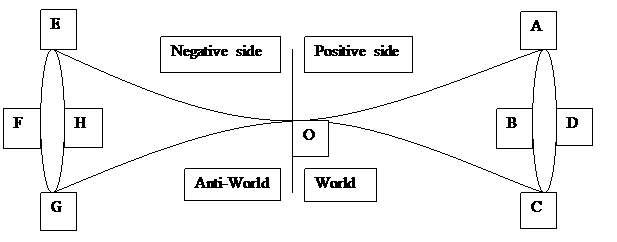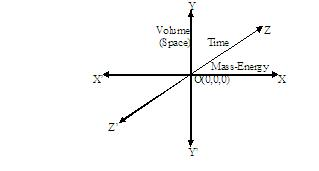A New Concept of the UniverseBasic Assumptions:- 1. There are three fundamental things- For positive side- i) Matter-Energy(Mass-Energy) ii) Positive Volume(Space) iii) Positive Time For negative side- i) Anti-Matter-Anti-Energy(Mass-Energy) ii) Negative Volume(Space) iii) Negative Time 2. At the point O everything is zero, i.e. there was nothing at this point. 3. Everything is symmetric in the two sides. 4. World + Anti-World = Universe Theory:- Time has an intention to go apart from O. It also tends to carry mass-energy and volume(space) with it. But mass-energy does not want to move with time. Greater mass-energy creates greater objection to move with time. Because, there is always an attraction between the anti-matter-anti-energy of the anti-world and matter-energy of the world. Hence they always want to meet at O. Greater mass-energy makes greater curve in the time-field. In this curve time becomes slower than other places. Hence smaller mass-energy tends to come here, so that the distance between the anti-matter-anti-energy and the matter-energy may become shorter. Here the Gravitational Force of Attraction appears. When a very large mass-energy is created in the positive side, the same but opposite thing is also created in the negative side. The attraction between them may stop the progress of time in their place. The curves made by them may touch or may increase to touch each other at O. This very curve is called Black-Hole. When the curves of positive and negative sides touch each other, an Warm-Hole is established. This Warm-Hole can be used as Time-Machine. Through this hole we may go to any time of the past. But a body of positive side can not go to the negative side of the world, because, if they touch each other, they will become zero, i.e. they will be annihilated. Hence, if the positive and negative sides collide, there will be nothing. In each sides of the world (i.e. positive and negative sides), there are oppositely charged particles, which create the two sides of the universe. Hence the Electro-Magnetic Force of Attraction is active separately in the two sides of the universe. Strong and Weak Nuclear Forces are also active separately in the two sides. Electro-Magnetic Force, Strong and Weak Nuclear Forces are active only in one Time-Circle (like ABCD or EFGH). They have no connection with any other Time-Circle in the same side. But the three forces, mentioned just above, will act in the same manner in the same Time-Circles at the two sides of the universe (like ABCD or EFGH). These three forces are inter-related, but independent of Force of Gravitation. Force of Gravitation is dependent on time. Here, it may be mentioned that, at any instant, we can see all the happenings of only one Time-Circle. Conclusion:- I think, to get the actual picture of the universe, we have to proceed in the following way - 1. We have to find the 3-dimensional graph of the three variables - i) Mass-Energy ii) Volume(Space) iii) Time The co-ordinates will be as under -To find the graph, the points given under, should be followed - i) Same unit should be invented for Mass-Energy. ii) Volume should be measured under 0 Kelvin temperature and 0 atmospheric pressure. (There will be no existence of gas. Hence volume can not be unlimited.) iii) If the positive and negative world come in contact, the resultant will come to the point O(0,0,0). 2. We have to invent the mathematical equation between the relations given below - i) Time in both sides is directly proportional to Mass-Energy. ii) Time in both sides is directly proportional to Volume(Space). Please enable JavaScript to view the comments powered by Disqus. comments powered by Disqus <= More Articles